The Data Encryption Standard (DES) is a symmetric-key block cipher published by the National Institute of Standards and Technology (NIST).

DES is an implementation of a Feistel Cipher. It uses 16 round Feistel structure. The block size is 64-bit. Though, key length is 64-bit, DES has an effective key length of 56 bits, since 8 of the 64 bits of the key are not used by the encryption algorithm (function as check bits only). General Structure of DES is depicted in the following illustration −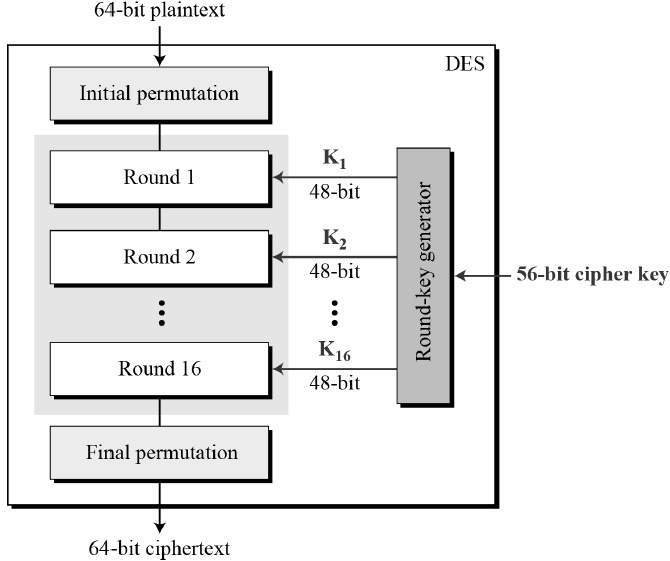Since DES is based on the Feistel Cipher, all that is required to specify DES is −

• Round function
• Key schedule
• Any additional processing − Initial and final permutation

## Initial and Final Permutation

The initial and final permutations are straight Permutation boxes (P-boxes) that are inverses of each other. They have no cryptography significance in DES. The initial and final permutations are shown as follows −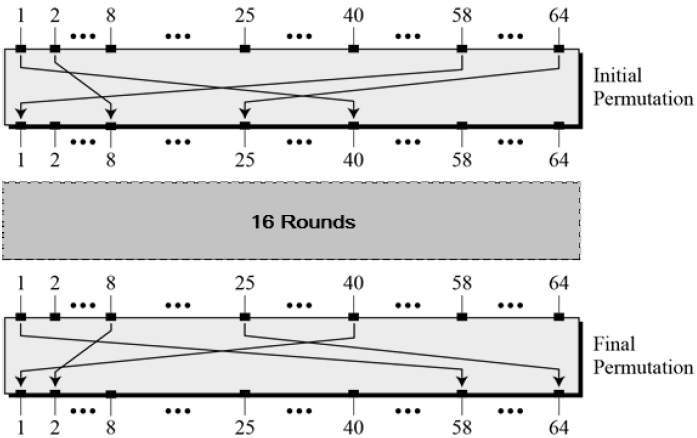## Round Function

The heart of this cipher is the DES function, f. The DES function applies a 48-bit key to the rightmost 32 bits to produce a 32-bit output.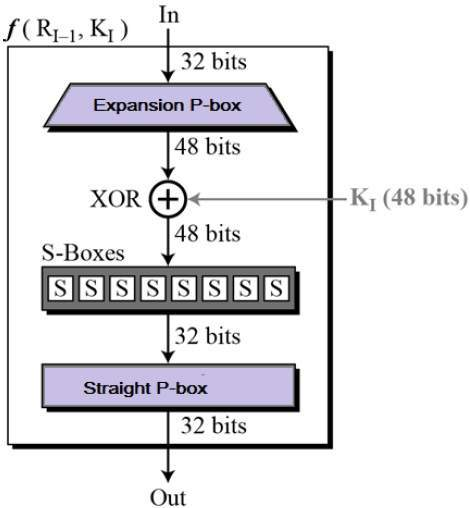• Expansion Permutation Box − Since right input is 32-bit and round key is a 48-bit, we first need to expand right input to 48 bits. Permutation logic is graphically depicted in the following illustration −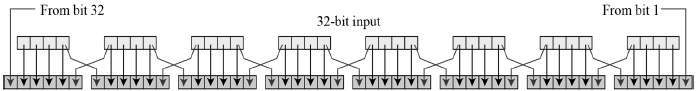• The graphically depicted permutation logic is generally described as table in DES specification illustrated as shown −• XOR (Whitener). − After the expansion permutation, DES does XOR operation on the expanded right section and the round key. The round key is used only in this operation.

• Substitution Boxes. − The S-boxes carry out the real mixing (confusion). DES uses 8 S-boxes, each with a 6-bit input and a 4-bit output. Refer the following illustration −• The S-box rule is illustrated below −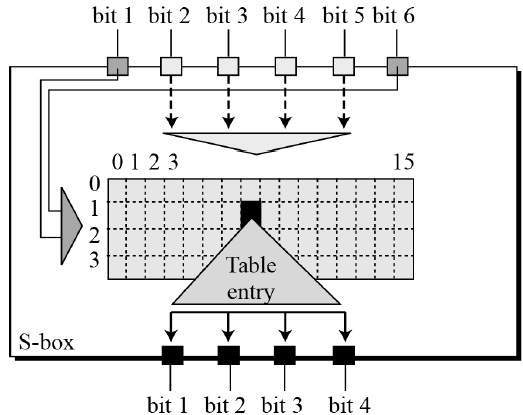• There are a total of eight S-box tables. The output of all eight s-boxes is then combined in to 32 bit section.

• Straight Permutation − The 32 bit output of S-boxes is then subjected to the straight permutation with rule shown in the following illustration:## Key Generation

The round-key generator creates sixteen 48-bit keys out of a 56-bit cipher key. The process of key generation is depicted in the following illustration −The logic for Parity drop, shifting, and Compression P-box is given in the DES description.

## DES Analysis

The DES satisfies both the desired properties of block cipher. These two properties make cipher very strong.

• Avalanche effect − A small change in plaintext results in the very grate change in the ciphertext.

• Completeness − Each bit of ciphertext depends on many bits of plaintext.

During the last few years, cryptanalysis have found some weaknesses in DES when key selected are weak keys. These keys shall be avoided.

DES has proved to be a very well designed block cipher. There have been no significant cryptanalytic attacks on DES other than exhaustive key search.